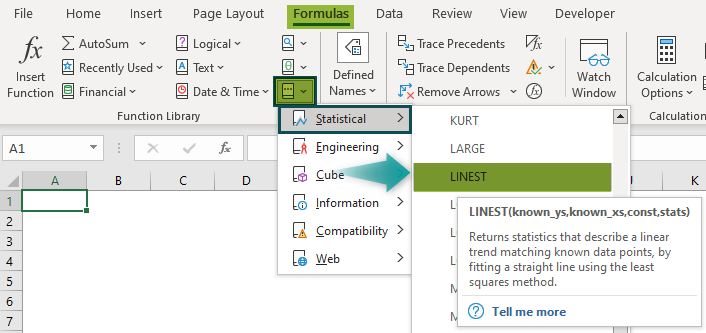# LINEST Excel FunctionArticle byExcelMojo Team## What Is LINEST Function In Excel?

The LINEST function in Excel generates regression statistics for a linear regression model. It is an array formula that can be used alone or with other functions to calculate specific statistics about the model. It is an inbuilt function, a part of the Statistical functions in the Function Library.

For example, the image below shows two sets of values in columns A and B. We will find the output using the LINEST function in Excel. In cell B5, enter the formula =LINEST(A2:A4,B2:B4,FALSE,FALSE), and press the “Enter” key.

[Note: Ignore the @ symbol after the = sign in the formula, it is available in some Excel versions].

The output is 0.31707, as shown above.

###### Key Takeaways
• The LINEST Excel function helps us to calculate the regression with the help of the known values as input.
• The ‘y’-intercept values can directly be calculated by using an independent x-variable, slope, and also with the following formulas:
• slope: =INDEX(LINEST (known_y’s,known_x’s),1)
• y-intercept: =INDEX(LINEST(known_y’s,known_x’s),2)
• On entering an array constant “known_xs” argument, use commas to separate the values in the same row and semicolons to separate the rows. Separator characters may vary depending on native regional settings.
• The value of ‘y’ predicted by the regression equation may not be valid if they are outside the range of the ‘y’ values used to determine the equation.
• We can describe a straight line with slope and y-intercept:
• Slope (m): The ‘m’ represents the slope of a line. For a line with two points (x1, y1) and (x2, y2); the slope is calculated as m=(y2 – y1)/(x2 – x1).
• Y-intercept (b): The ‘b’ representing the y-intercept of a line is the value of y at the point where the line crosses the y-axis.
• The equation of a straight line is “y = mx + b”. Once the values of m and b are known, we can calculate any point on the line by putting the ‘y’ or ‘x’ value into the equation.

### LINEST Excel Formula

The Syntax of the LINEST function in Excel formula is,

The arguments of the LINEST function in Excel formula are,

Note: If the range of “known_ys” is in a single column, each column of “known_xs” is interpreted as a separate variable. Similarly, if the range of “known_ys” is in a single row, each row of “known_xs” is interpreted as a different variable.

• known_ys: It indicates the set of y-values already known in the relationship “y = mx + b”. It is a mandatory argument.
• known_xs: It indicates the set of x-values already known in the relationship “y = mx + b”. It is an optional argument.
• const: It represents a logical value (TRUE/FALSE) specifying whether to force the constant ‘b’ to be equal to ‘0’. It is an optional argument.
• stats: It represents a logical value (TRUE/FALSE) specifying whether to return additional regression statistics. It is an optional argument.

The range of “known_xs” can have one or more sets of variables. When only one variable is used, the “known_ys” and the “known_xs” can be the ranges of any shape with equal dimensions. If more than one variable is used, “known_ys” must be a vector i.e., a range with a row height or column width.

Whenever the value of the “const” argument is TRUE or skipped, ‘b’ is calculated normally, but if the value of the “const” argument is FALSE, ‘b’ is set as equal to 0, and the values of ‘m’ are adjusted to fit the formula “y = mx”.

When the value of the “stats” argument is TRUE, the LINEST Excel Function returns the additional regression statistics. And if the value of the “stats” argument is FALSE or skipped, LINEST Excel Function returns only the ‘m’ coefficients and the constant ‘b’.

### How To Use LINEST Excel Function?

We can use the LINEST Excel Function in 2 methods, namely,

1. Access from the Excel ribbon.
2. Enter the worksheet manually.

#### #Method 1 – Access from the Excel ribbon

Choose an empty cell for the output 🡪 select the “Formulas” tab 🡪 go to the “Functions Library” group 🡪 click the “More Functions” drop-down 🡪 click the “Statistical” right-arrow 🡪 click the “LINEST” function, as shown below.

The formula is inserted in the cell, and instantly the “Function Arguments” window opens, where we can enter the values or the Excel cell references in the “Known_ys”, “Known_xs”, “Const”, and “Stats” fields accordingly and click “OK.

#### #Method 2 – Enter the worksheet manually

1. Select an empty cell for the output.
2. Type =LINEST( in the selected cell. [Alternatively, type “=L” and double-click the LINEST function from the list of suggestions shown by Excel].
3. Enter the arguments as cell references or direct values.
4. Close the parenthesis and press the “Enter” key.

# Basic Example

We will calculate the regression using LINEST function in Excel for the x and y values.

In the following table, the data is,

• Column A contains the ‘x’ values.
• Column B contains the ‘y’ values.

The steps to calculate the regression using LINEST Excel Function are as follows:

1. Select cell B7 and enter the formula =Linest(B2:B6, i.e., the known_ys argument.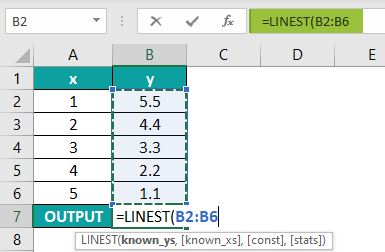2. In continuation with the formula, select the cell range A2:A6 i.e., the known_xs argument.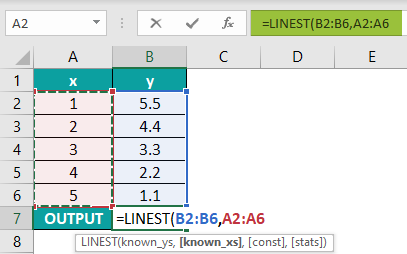3. Enter the “const” argument as “TRUE” in continuation with the formula, which means the value of ‘b’ is calculated normally.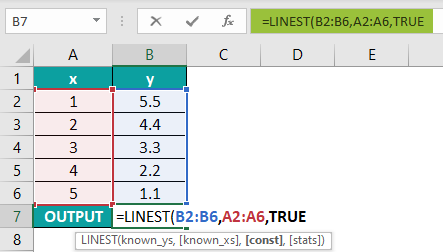4. Enter the “stats” argument as “TRUE”, in continuation with the formula, which means the value returns additional regression statistics.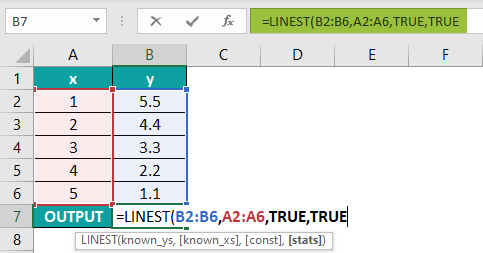5. Press the “Enter” key. The complete formula is =LINEST(B2:B6,A2:A6,TRUE,TRUE).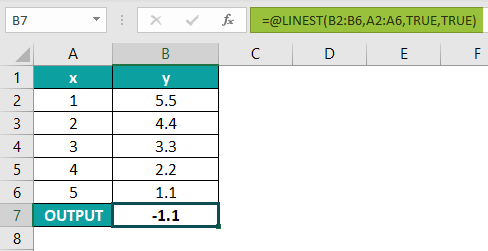The output is “-1.1”, as shown above.

### Examples

We will understand the LINEST Excel Function with some advanced scenarios

#### Example#1

We will calculate the regression using the LINEST function in Excel with a line graph.

In the following table, the data is,

• Column A contains the ‘x’ values.
• Column B contains the ‘y’ values.

The steps to calculate the regression using LINEST Excel Function with a line graph are as follows:

Step 1: Select cell B7 for the output.

Step 2: Enter the complete formula =LINEST(B2:B6,A2:A6,TRUE,TRUE) and press the “Enter” key. The calculated result is “0.0773” in cell B7, as shown below.

[Note: Ignore the @ symbol after the = sign in the formula, it is available in some Excel versions].

Step 3: To graphically view the values, select the cell range A2:B6 🡪 go to the “Insert” tab 🡪 go to the “Charts” group 🡪 click the “Insert Line or Area Chart” drop-down 🡪 select the “Line” chart under the “2-D Line” group, as shown below. Instantly a chart is created, as seen behind the selections.

Step 4: To format the chart, click on the chart 🡪 the “Chart Design” tab appears on the ribbon 🡪 select the “Chart Design” tab 🡪 go to the “Chart Styles” group 🡪 select the desired format, as shown below.

The final output with the line chart is shown above.

#### Example#2

We will calculate the output using the LINEST Excel function for the monthly sales and quantity.

In the following table, the data is,

• Column A contains the Months.
• Column B contains the Sales.
• Column C contains the Quantity.

The steps to calculate the regression using the LINEST Excel function are as follows:

Step 1: Select cell C14 for the output.

Step 2: Enter the formula =LINEST(B2:B13,C2:C13) and press the “Enter” key.

The output is “1.69127”, as shown above. It is calculated without “const” and “stats” arguments.

#### Example#3

We will calculate the output using the LINEST Excel function for the time and distance values.

In the following table, the data is,

• Column A contains the Time in Seconds.
• Column B contains the Distance in Meters.

The steps to calculate the regression using the LINEST Excel function are as follows:

Step 1: Select cell B6 for the output.

Step 2: Enter the formula =LINEST(A2:A5,B2:B5,FALSE,FALSE) and press the “Enter” key.

The output is “3.8490566”, as shown above.

### LINEST Function Not Working

The LINEST function in Excel will not work due to the following reasons;

• We get the #VALUE! error,
• If the “known_xs” or “known_ys” values contain at least one blank cell, text value, or a text representation of a number that Excel does not recognize as a numeric value.
• If the “const” or “stats” arguments cannot be evaluated to TRUE or FALSE.
• The #REF! error occurs if the “known_xs” and “known_ys” ranges have different dimensions.
• The LINEST Excel Function returns only a “slope coefficient” number because we have entered it as a regular formula, not an array formula. To correct the formula, press the shortcut keys CTRL+SHIFT+ENTER, then the formula changes to array format represented within the curly brackets “{}” which is visible in the formula bar.

What does the LINEST function do in Excel?

The LINEST Excel function is a linear regression function that predicts data following a straight line. Linear Regression predicts values such as sales growth, inventory requirements, or simple market trends.

To achieve similar outcomes, the function performs the Forecasting but with far more information about the regression model and uses more than one independent variable.

LINEST function syntax of the is =LINEST(known_ys,known_xs,const,stats).

How to enter the LINEST function in Excel?

We can enter the LINEST function in Excel formula as follows:

● Select an empty cell for the output.
● Type =LINEST( or type =L and double-click the LINEST function from the list of suggestions shown by Excel.
● Enter the cell reference values.
● Close the brackets ) and press the “Enter” key.

The following image shows two sets of values in columns A and B.

In cell B5, enter the formula =LINEST(A2:A4,B2:B4,TRUE,TRUE) and press the “Enter” key.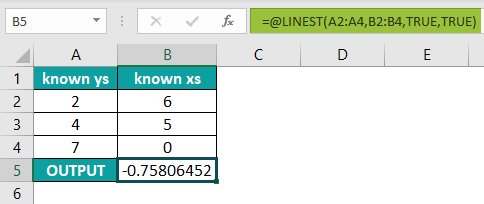The output is ‘-0.75806452’, as shown above.

Where is the LINEST function in Excel?

The Linest Excel function is used as follows:

Choose an empty cell for the output 🡪 select the “Formulas” tab 🡪 go to the “Functions Library” group 🡪 click the “More Functions” drop-down 🡪 click the “Statistical” right-arrow 🡪 click the “LINEST” function, as shown below.